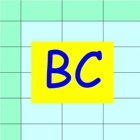Boyle Charles Calculator

• SEARCH TYPE

All iPhone applications categories

All iPhone games categories# Boyle Charles Calculator

0 0

## Screenshots

Description

Boyle Charles Calculator is a calculation sheet that solves a gas problem of volume, pressure, and temperature. This Boyle Charles Calculator is a subset of iPad Mol Calculator software.

A typical problem is as follows.

(Example)
There is 0.05 mol of hydrogen gas.
(1)When the temperature is 20 ℃ and the pressure is 1 atmosphere, how much liters is the gas ?
(2)When the temperature is 20 ℃ and the gas is packed in a 0.1-liter container, how much is the pressure of hydrogen gas?

(1)Volume calculation

Boyle Charles Calculator solves the problem in the following three steps.

//Step 0: Preparation//
Gather known data.

//Step 1: Definition//
Enter known data into the BC sheet.
Mol = 0.05, T = 20[C], p = 1[atm]

//Step 2: Calculation//
Data are not independent but mutually related by formulae.
Calculation is a conversion from unknown data to known data using the formulae.
The formulae are embedded in P and G buttons.
Touching out those buttons executes the formulae and changes unknown data to known data.
Touch out buttons as follows.
Touch out P_Mol button. StdVol and Vol data are changed to known data.
It turns out that the volume of hydrogen gas is 1.2 L.

(1)Pressure calculation

//Solution by V1.00//
Use the above results.
Enter the following data
Vol = 0.1[L]
Touch out G_Pres button. Pressure data are changed to known data.
It turns out that the gas pressure is 12.02 atmospheres.

//Solution by V2.00//
Change PresVol mode to pressure mode.
//Step 1: Definition//
Enter the following data.
Mol = 0.05, T = 20[C], Vol = 0.1[L]

//Step 2: Calculation//
Touch out P_Mol button. StdVol and Pres data are changed to known data.
It turns out that the gas pressure is 12.02 atmospheres.

[Data label]
n [mol]: Mol quantity of substance.
StdVol [L]: Gas volume of substance at 0 deg C and 1 atm.
T [C][F]: Temperature.
p [atm][kPa][mmHg]: Pressure
Vol [L]: Gas volume of substance at the temperature T and at the pressure p.

[Buttons and data]
//P button and P data group//
P (put) button inputs data to which the P button is assigned.
P data group is a group of data to which the P buttons are assigned. The P data group has a special character. When one P data is input, all other data of the P data group are updated by formulae.

//G button//
G (get) button gets data from other data using formulae.

//C butto//
C (Clear) button clears all data.

//PresVol button//
Either pressure data or volume data belongs to P button data group.
The PresVol button specifies pressure mode or volume mode.

[Color change]
Color change shows what data are input, output, or referenced data in this button execution.
Input data:Blue color
Referenced data:Yellow color
Output data:Green color
Uncalculated data:Red color

[Formulae]
Embedded formulae are as follows.
StdVol = 22.414 x n
StdVol /273.15 = p x Vol / (T + 273.15)